# CBSE Class 11 Economics Organisation of Data Worksheet Set C

Download printable Economics Class 11 Worksheets in pdf format, CBSE Class 11 Economics Organisation of Data Worksheet Set C has been prepared as per the latest syllabus and exam pattern issued by CBSE, NCERT and KVS. Also download free pdf Economics Class 11 Assignments and practice them daily to get better marks in tests and exams for Grade 11. Free chapter wise worksheets with answers have been designed by Standard 11 teachers as per latest examination pattern

CHAPTER 3 ORGANISATION OF DATA

INTRODUCTION

The purpose of classifying raw data is to bring order in them so that they can be subjected to further statistical analysis easily. While classification of objects or things saves our valuable time and effort, it is not done in a random manner. Classification, therefore, is arranging or organising similar things into groups or classes.

RAW DATA

Unclassified data or highly disorganised data is raw data. They are often very large and difficult to handle. To draw meaningful conclusions from them, proper organisation and presentation of such data is needed before any systematic statistical analysis is undertaken. Hence after collecting data the next step is to organise and present them in a classified form as presented as a table, diagrams and graphs.

CLASSIFICATION OF DATA

The raw data are summarised, and made comprehensible by classification. When facts of similar characteristics are placed in the same class, it enables one to locate them easily, make comparison, and draw inferences without any difficulty.

The groups or classes of a classification can be done in various ways.

• Chronological Classification: Raw data classified according to time is known as a Chronological Classification. In such a classification, data are classified either in ascending or in descending order with reference to time such as years, quarters, months, weeks, etc.

• Spatial Classification: In Spatial Classification the data are classified with reference to geographical locations such as countries, states, cities, districts, etc.

Qualitative Classification: Data classified according to characteristics that cannot be quantified. For example, nationality, literacy, religion, gender, marital status, etc. They cannot be measured. Yet these attributes can be classified on the basis of either the presence or the absence of a qualitative characteristic.

• Quantitative Classification: data that can be quantified or expressed in numerical values. Characteristics like height, weight, age, income, marks of students, etc. are quantitative in nature.

VARIABLES: CONTINUOUS AND DISCRETE

Different variables vary differently. They are broadly classified as:
1. Continuous and
2. Discrete.

A continuous variable can take any numerical value. It m,y take integral values (1, 2, 3, 4,), fractional values (1/2, 2/3, 3/4, ), and values that are not exact fractions Other examples of a continuous variable are weight, time, distance, etc.
A discrete variable can take only certain values. Its value changes only by finite "jumps". For example, a variable like the "number of students in a class", for different classes, would assume values that are only whole numbers. It cannot take any fractional value like 0.5 because "half of a student" is does not make sense.

♦ WHAT IS A FREQUENCY DISTRIBUTION?

A frequency distribution is a comprehensive way to classify raw data of a quantitative variable. It shows how the different values of a variable (here, the marks in mathematics scored by a student) are distributed in different classes along with their corresponding class frequencies.

Class Frequency means the number of values in a particular class. Each class in a frequency distribution table is rounded by Class Limits. Class limits are the two ends of a class. The lowest value is called the Lower Class Limit and the highest value the Upper Class Limit.

The Class Mid-Point or Class Mark is the middle value of a class. It lies halfway between the lower class limit and the upper class limit of a class and can be ascertained in the following manner: Class Mid-Point or Class Mark = (Upper Class Limit + Lower Class Limit) /2

Example of frequency distribution♦ HOW TO PREPARE A FREQUENCY DISTRIBUTION?

While preparing a frequency distribution, the following four questions need to be kept in mind:

1. How many classes should we have?
2. What should be the size of each class?
3. How should we determine the class limits?
4. How should we get the frequency for each class?

♦ How many classes should we have?

Before determining the number of classes, it is necessary to find out the extent the variable in hand changes in value. It is called the range of classes and it is the difference between the largest and the smallest values of the variable. A large range indicates that the values of the variable are widely spread. A small range indicates that the values of the variable are spread narrowly. After obtaining the value of range, it becomes easier to determine the number of classes once the number of class interval is known. Given the value of range, the number of classes would be large if we choose small class intervals. A frequency distribution with too many classes would look too large. Such a distribution is not easy to handle. Similarly, if we choose a class interval that is too large then the number of classes becomes too small. Though there is no hard-and-fast rule to determine the number of classes, the rule of thumb often used is that the number of classes should be between 5 and 15.

♦ What should be the size of each class?

The answer to this question depends on the answer to the previous question. We can determine the number of classes once we decide the class interval. Similarly, we can determine the class interval once we decide the number of classes. Thus we find that these two decisions are inter-linked with one another. We cannot decide on one without deciding on the other.

♦ How should we determine the class limits?

If 0 is the lower class limit of the first class: 0-10, It is because we chose the minimum value of the variable as the lower limit of the first class. Similarly, for the upper class limit for the last class is a

value greater than the maximum value of the variable. The class limits must be such chosen that the midpoint or class mark of each class coincide, as far as possible, with any value around which the data tend to be concentrated.

♦ There are two different methods of class limits are:

Exclusive Method: The classes, by this method, are formed in such a way that the upper class limit of one class equals the lower class limit of the next class. In this way the continuity of the data is maintained. That is why this method of classification is most suitable in case of data of a continuous variable. Under this method, the upper class limit is excluded but the lower class limit of a class is included in the interval. Therefore, any observation that is exactly equal to the upper class limit, according to the method, would not be included in that class but would be included in the next class. On the other hand, if it were equal to the lower class limit then it be included in that class.

Inclusive Method: In comparison to the exclusive method, the Inclusive Method does not exclude the upper class limit in a class interval. It includes the upper class in a class. Thus both class limits are parts of the class interval.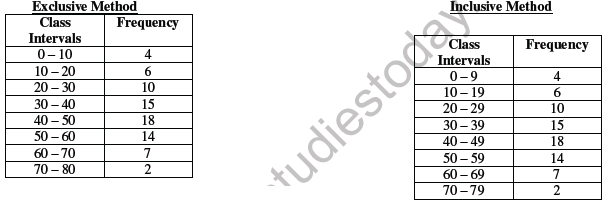♦ How should we get the frequency for each class?

In simple terms, frequency of an observation means how many times that observation occurs in the raw data. Class Frequency refers to the number of values in a particular class. The counting of class frequency is done by tally marks against the particular class. A tally ( I ) is put against an observation. The counting of tally is made easier when four of them are put as ( IIII ) and the fifth tally is placed across then as ( / ). This method is more commonly known as the “four and cross method” . Thus frequency in a class is equal to the number of tallies against that class.

LOSS OF INFORMATION

The classification of data as a frequency distribution has an inherent shortcoming. While it summarises the raw data making it concise and comprehensible, it does not show the details that are found in raw data. There is a loss of information in classifying raw data. Once the data are grouped into classes, an individual observation has no significance in further statistical calculations. For Example, the class 20-30 contains 6 observations: 25, 25, 20, 22, 25 and 28. So when these data are grouped as a class 20-30 in the frequency distribution, it provides only the number of records in that class and not their actual values. All values in this class are assumed to be equal to the middle value of the class interval Statistical calculations are based only on the values of class mark and not on the values of the observations in that class. Thus the use of class mark instead of the actual values of the observations in
statistical methods involves considerable loss of information.

→ FREQUENCY DISTRIBUTION WITH UNEQUAL CLASSES

In some cases frequency distributions with unequal class intervals are more appropriate. This is done when most of the frequencies are concentrated around some classes and are widely spread for other classes.

Example of unequal frequency distributionIn the above frequency distribution, observations 15, 18, 14, 10 and 9 are concentrated in the classes 0 – 10, 10 – 20, 20 – 30, 40 – 50. The remaining frequencies 4, 2, 1 are in the classes 50 – 70, 70 – 90, and 90 – 120. These classes are thinly populated with observations.

♦  FREQUENCY ARRAY

For a discrete variable, the classification of its data is known as a Frequency Array. Since a discrete variable takes values and not intermediate fractional values between two integral values, we have frequencies that correspond to each of its integral values.

Frequency Array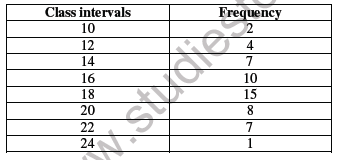BIVARIATE FREQUENCY DISTRIBUTION

A Bivariate Frequency Distribution is the frequency distribution of two variables.

CONCLUSION

The data collected from primary and secondary sources are raw or unclassified. Once the data is collected, the next step is to classify then for further statistical analysis. Classification brings order in the data. Data can be classified through a frequency distribution in a comprehensive manner.

EXERCISES

1. Define raw data

2. What are the different ways of classification of data? Explain

3. What is a frequency distribution?

4. Distinguish between “exclusive method” and “inclusive method”

5. What are steps involved in the construction of a frequency distribution?

6. What is a variable? Distinguish between a discrete and a continuous variable.

7. What is loss of information in classified data?

8. Do you agree that classified data is better than raw data?

9. Distinguish between uni-variate and bi-variate frequency distribution.

10. Prepare a frequency distribution by inclusive method taking class interval of 7 from the following data: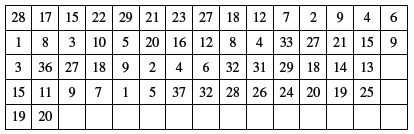11. Prepare a frequency distribution from the following data using a appropriate class interval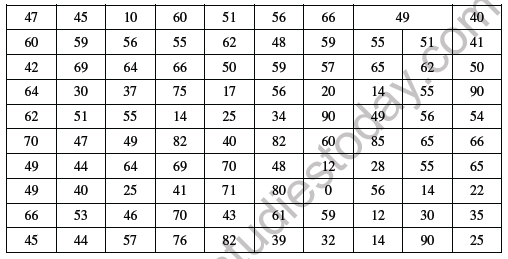12. Prepare a frequency distribution from the following data using a appropriate class interval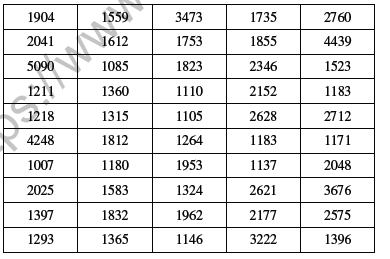## Tags:

Click for more Economics Study Material
 CBSE Class 11 Micro Economics Consumer Behaviour And Demand Utility Analysis Worksheet CBSE Class 11 Economics Elasticity of Demand Worksheet CBSE Class 11 Economics Utility Analysis Worksheet CBSE Class 11 Micro Economics Consumer Behaviour And Demand Analysis Worksheet CBSE Class 11 Economics On Median And Quartiles Worksheet CBSE Class 11 Economics Arithmetic Mean And Weighted Mean Worksheet CBSE Class 11 Economics Statics For Economics Worksheet Set A CBSE Class 11 Economics Introduction Worksheet CBSE Class 11 Economics Worksheet Set G Solved CBSE Class 11 Economics Organisation of Data Worksheet Set C CBSE Class 11 Economics Indefference Curve Analysis Worksheet CBSE Class 11 Economics Worksheet Set A Solved CBSE Class 11 Micro Economics Consumer Behaviour And Demand Indifference Analysis Worksheet Set A CBSE Class 11 Economics On Poverty Worksheet CBSE Class 11 Economics Collection of Data Worksheet Set A CBSE Class 11 Economics Statics For Economics Worksheet Set B CBSE Class 11 Economics Market Equilibrium Worksheet CBSE Class 11 Economics Worksheet Set H Solved CBSE Class 11 Economics Organisation of Data Worksheet Set D CBSE Class 11 Economics Index Number Worksheet CBSE Class 11 Economics Worksheet Set B Solved CBSE Class 11 Micro Economics Indifference Curve Analysis Worksheet CBSE Class 11 Economics On Presentation of Data Worksheet CBSE Class 11 Economics Collection of Data Worksheet Set B CBSE Class 11 Economics Statics For Economics Worksheet Set C CBSE Class 11 Economics Median And Quartiles Worksheet CBSE Class 11 Economics Worksheet Set I Solved CBSE Class 11 Economics Presentation of data Worksheet CBSE Class 11 Economics Indian Economy On The Eve Of Independence Worksheet CBSE Class 11 Economics Worksheet Set C Solved CBSE Class 11 Economics On Range and Quartile Deviation Worksheet CBSE Class 11 Micro Economics Introduction Worksheet CBSE Class 11 Economics Collection of Data Worksheet Set C CBSE Class 11 Economics Statics Introduction Worksheet CBSE Class 11 Economics Non Competitive Market Worksheet CBSE Class 11 Economics Worksheet Set J Solved CBSE Class 11 Economics Production And Cost Worksheet CBSE Class 11 Economics Indian Economy Worksheet CBSE Class 11 Economics Worksheet Set D Solved CBSE Class 11 Economics On Rural Development Worksheet CBSE Class 11 Micro Economics Price Elasticity of Demand Worksheet CBSE Class 11 Economics Comparative Development Experience Worksheet CBSE Class 11 Economics Statistics For Economics Worksheet CBSE Class 11 Economics On Employment Worksheet CBSE Class 11 Economics Worksheet Set K Solved CBSE Class 11 Economics Production Function Worksheet CBSE Class 11 Economics Introduction to Microeconomics Worksheet CBSE Class 11 Economics Worksheet Set E Solved CBSE Class 11 Economics Organisation of Data Worksheet Set A CBSE Class 11 Micro Economics Theory of Demand Worksheet CBSE Class 11 Economics Demand Analysis Worksheet Set A CBSE Class 11 Economics Theory of Firm Under Perfect Competition Worksheet CBSE Class 11 Economics Worksheet Set L Solved CBSE Class 11 Economics On Human Capital Formation Worksheet CBSE Class 11 Economics Production Possibility Curve Worksheet CBSE Class 11 Economics Introduction Worksheet Set A CBSE Class 11 Economics Worksheet Set F Solved CBSE Class 11 Economics Organisation of Data Worksheet Set B

## Latest NCERT & CBSE News

Read the latest news and announcements from NCERT and CBSE below. Important updates relating to your studies which will help you to keep yourself updated with latest happenings in school level education. Keep yourself updated with all latest news and also read articles from teachers which will help you to improve your studies, increase motivation level and promote faster learning

### Score well in Class 12 English Boards Exam

12th Board exams are an important part of students' lives. The marks obtained in the board exam decide the college in which one can study. In class 12 the syllabus of each and every subject increases vastly and it is difficult to cover up every point. In English also...

### CBSE Class 10 Revised Syllabus

Last year CBSE had to reduce the syllabus because of the pandemic situation but it was not very effective because there were no examinations. This year to avoid any confusion and conflict, CBSE has decided to reduce the syllabus into term 1 and term 2. 50 percent of...

### Moderation of Marks Class 11 and 12 Board Exams

The portal for moderation and finalization of results for Class-12 is being opened from 16.07.2021 to 22.07.2021. As Board has to declare the result latest by 31.07.2021, schools have been requested to follow the schedule strictly and complete the moderation within...

### CBSE Board Examination Scheme of Assessment 2021 2022

A. Academic session to be divided into 2 Terms with approximately 50% syllabus in each term: The syllabus for the Academic session 2021-22 will be divided into 2 terms by following a systematic approach by looking into the interconnectivity of concepts and topics by...

### MCQ Question based CBSE examination

For 2021-22 CBSE has launched MCQ question-based examination for Term 1 & Term 2 board examinations. The entire syllabus has been divided into two parts each including 50% of the entire syllabus. To score well, students must practice as per the new CBSE term-wise...

### How To Solve Unseen Passages In English

Unseen passages may contain one or many paragraphs. This is one of the important yet easy parts for a student to get marks. Students should thoroughly study and understand the passage to answer the related questions. The unseen passages are there just to test the...

×